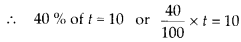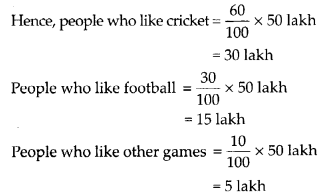# MP Board Class 8th Maths Solutions Chapter 8 Comparing Quantities Ex 8.1

## MP Board Class 8th Maths Solutions Chapter 8 Comparing Quantities Ex 8.1

Question 1.
Find the ratio of the following.
(a) Speed of a cycle 15 km per hour to the speed of scooter 30 km per hour.
(b) 5 m to 10 km.
(c) 50 paise to ₹ 5.
Solution:
(a) The ratio is 15 km/hr : 30 km/hr or 1 : 2.
(b) The ratio is 5 m : 10 km
or 5 m : 10 × 10 m (∵ 1 km = 103 m)
or 1 : 2000.
(c) The ratio is 50 paise : ₹ 5
or 50 paise : 500 paise
( ∵ ₹1 = 100 paise)
or 1 : 10.Question 2.
Convert the following ratios to percentages.
(a) 3 : 4
(b) 2 : 3
Solution:
To convert a ratio to its percentage counterpart, we multiply it by 100.
Thus,
(a) Ratio = 3 : 4
and percentage = $$\frac{3}{4}$$ × 100 = 75 %
Ratio = 2 : 3
and percentage = $$\frac{2}{3}$$ × 100 = 66$$\frac{2}{3}$$ %

Question 3.
72% of 25 students are good in mathematics. How many are not good in mathematics?
Solution:
We have, 72% of 25 students are good in Mathematics.
∴ percentage of students not good in Mathematics = 100% – 72% = 28%
Also, total number of students = 25
Hence, the number of students not good in
Mathematics = $$\frac{28}{100} \times 25=7$$Question 4.
A football team won 10 matches out of the total number of matches they played. If their win
percentage was 40, then how many matches did they play in all?
Solution:
Number of matches won by football
team = 10
Win % = 40
Let total number of matches = tor t = 25
∴ They played 25 matches in all.Question 5.
If Chameli had ₹ 600 left after spending 75% of her money, how much did she have in the beginning?
Solution:
Money left with Chameli = ₹ 600 % of money she spent = 75 %
% of money left = 100% – 75% = 25%
Let total money in the beginning be x.
∴ 25 % of x = ₹ 600Question 6.
If 60% people in a city like cricket, 30% like football and the remaining like other games, then what percent of the people like other games? If the total number of people are 50 lakh, find the exact number who like each type of game.
Solution:
In the city,
People who like cricket = 60%
People who like football = 30%
People who like other games = 100% – (60 + 30) % = 10 %
Total population = 50 lakh